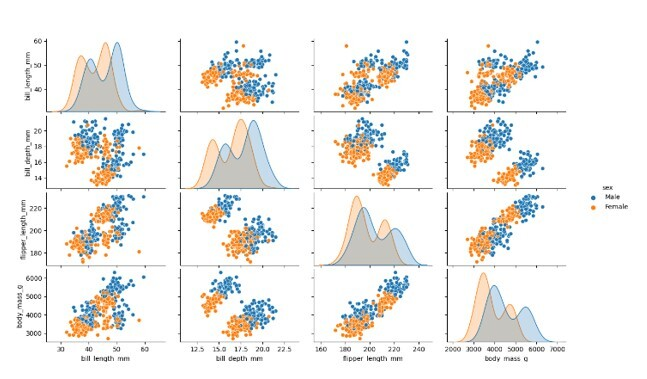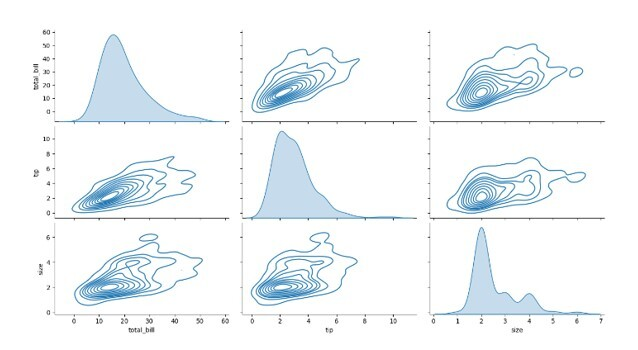# What is a Pairplot in Data Science?

The visual presentation of data is known as data visualization. Because of the excellent ecosystem of Python packages focused on data, it is crucial for data analysis. Summarising and presenting a large quantity of data in a straightforward and understandable style also helps to grasp the data, no matter how complicated it may be, as well as the value of the data. It also aids in the effective and clear transmission of information.

We may visualize pairwise connections between variables in a dataset using the Seaborn Pairplot. Condensing a lot of data into a single figure gives the data a pleasant visual representation and aids in our understanding of the data. This is crucial as we explore and become comfortable with our dataset.

When performing exploratory data analysis (EDA), pairplot visualisation is useful. The connection between the supplied data, where the variables may be continuous or categorical, is shown using a pairplot.

Plot pairwise relationships in a data-set.

The seaborn library's Pairplot module offers a high-level interface for creating visually appealing and educational statistics visuals.

## Importing Libraries and Data

Importing the libraries, we'll be using is the first step. In this instance, our data visualization framework will be Seaborn, and we'll import and save our data using the pandas programming language.

import seaborn as sns
import pandas as pd


## Syntax of the Seaborn Pairplot function

seaborn.pairplot(
data,
hue = None,
hue_order = None,
palette = None,
vars = None,
x_vars = None,
y_vars = None,
kind = 'scatter',
diag_kind = 'auto',
markers = None,
height = 2.5,
aspect = 1,
corner = False,
dropna = False,
plot_kws = None,
diag_kws = None,
grid_kws = None,
size = None
)


## Parameters of Pairplot function

• data − Depending on the visualization that will be shown, the data parameter accepts the data. A DataFrame, an Array, or a List of Arrays can represent the values.

• hue_order, order − The order of categorical variables used in the plot is determined by the hue order or order parameter. The lists of strings can be used as values for this parameter.

• scale − The plot is scaled using the scale option. Useful values for this property are area, count, and width.

• scale_hue − The scale hue option accepts a Boolean value to specify whether the scale is approximated across all violins on the plot for FALSE or within each level of the primary grouping variable for TRUE.

• gridsize − The gridsize parameter calculates the kernel density for the plot using an integer number.

• inner − The inner option lets users specify the violin plot's inner points. The options for this parameter are box, point, quartile, stick, or None.

• orient − The plot's orientation may be selected by the user using the orient option. Vertical or horizontal orientations are indicated by the letters "v" and "h," respectively.

• linewidth − The linewidth parameter determines the width of the grey lines used in the plot by taking a float integer as its value.

• color − The user may set the color range for each plot's data item using the color parameter. This parameter's value may be matplotlib color.

• palette − The palette parameter is used to specify the various shades of colors to be used for each level of the plot.

• axe − The axe option specifies the axes on which the plot will be built. This parameter's value may be matplotlib Axes.

### Example 1

# importing the required libraries
import seaborn as sbn
import matplotlib.pyplot as plt
# pairplot with the hue = gender parameter
sbn.pairplot(mydata, hue = 'gender')
# displaying the plot
plt.show()


### Output## Code Explanation

In the example above, we imported the necessary libraries and used the Seaborn load dataset() method to load the penguin data set to work with. The plot was then shown using the pairplot() method with the hue argument set to the value "gender." Finally, we have shown the plot to the viewers using the Matplotlib show() method. The pair plot was successfully created as a consequence.

### Example 2

# importing the required libraries
import seaborn as sbn
import matplotlib.pyplot as plt
# pairplot with the kind = kde parameter
sbn.pairplot(mydata, kind = 'kde')
# displaying the plot
plt.show()


### Output## Code Explanation

In the example above, we imported the necessary libraries and used the Seaborn load dataset() method to load the penguin data set to work with. The plot was then shown using the pairplot() method with the hue argument set to the value "gender." Finally, we have shown the plot to the viewers using the Matplotlib show() method. The pair plot was successfully created as a consequence.

## Conclusion

The Seaborn Pairplot is an excellent tool for data visualisation that aids in familiarising us with our data. On a single figure, we may plot a lot of data so that we can grasp it and acquire fresh ideas. A plot to have in your data science toolkit for sure. A powerful tool for quickly examining distributions and relationships in a dataset is the pair plot. Through the Pair Grid class, Seaborn offers a straightforward default method for creating pair plots that can be modified and expanded. A significant amount of the value in a data analysis project frequently comes from the plain display of data rather than the showy machine learning. A pairs plot is a fantastic place to start when conducting data analysis since it gives us a thorough initial view of our data.

Updated on: 05-May-2023

156 Views